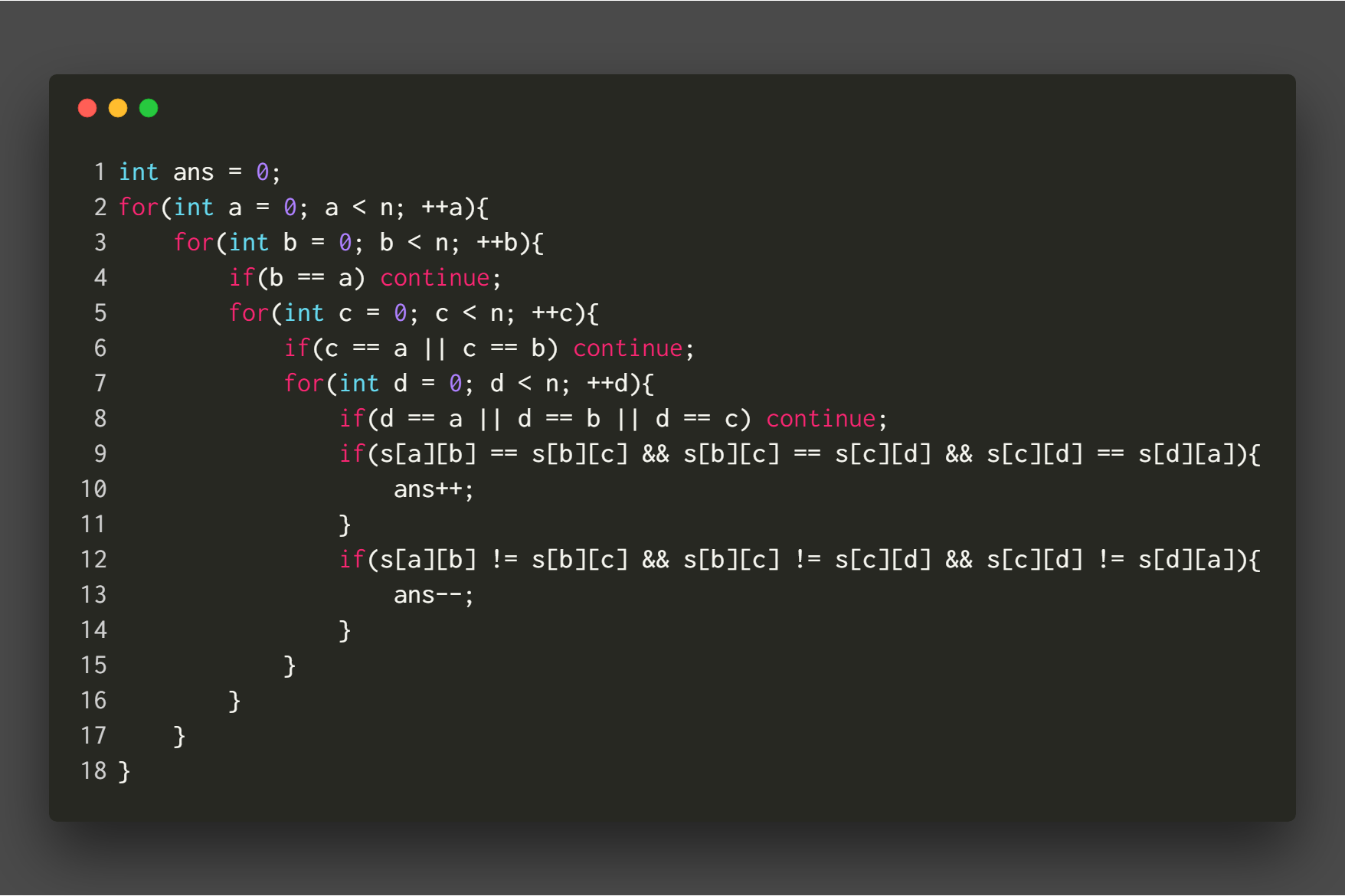F.A.Q
Hand In Hand
Online Acmers
Problem Archive
Realtime Judge Status
Authors Ranklist

C/C++/Java Exams
ACM Steps
Go to Job
Contest LiveCast
ICPC@China
Best Coder beta
VIP | STD Contests
DIY | Web-DIY betaAuthor ID Password
Register new ID

Time Limit: 2000/1000 MS (Java/Others)    Memory Limit: 65536/65536 K (Java/Others)
Total Submission(s): 156    Accepted Submission(s): 57

Problem Description
Given a tournament, you need to determine the direction of the remaining sides to maximize the answer. The answer is calculated in the following way. The vertices are labeled from $0$ to $n-1$, and the matrix $s$ is used to represent the edges.Input
The first line of input is a single line of integer $T (2 \le T \le 10)$, the number of test cases. In each test case, there are $1$ integers $n(5 \le n \le 200)$, denoting the number of vertices. Then in the following $n$ lines, the $i$-th line has a string of length $n$. If $s[i][j]=1$, there is an edge from $i$ to $j$. If $s[i][j]=2$ means you need to determine the direction for that edge. The input is guaranteed to be legal, and the number of $(i, j) (i < j)$ satisfying $s[i][j]=s[j][i]=2$ is less than $200$. The data is randomly generated.

Output
For each set of test samples, output one line to represent the maximized answer.

Sample Input
2
5
02112
20221
02001
02102
20020
5
01112
00022
01012
02002
22220

Sample Output
40
24

Hint

One solution to the first case is:
00110
10001
01001
01100
10010

Source

Statistic | Submit | Discuss | Note
 Home | Top Hangzhou Dianzi University Online Judge 3.0 Copyright © 2005-2023 HDU ACM Team. All Rights Reserved. Designer & Developer : Wang Rongtao LinLe GaoJie GanLu Total 0.000000(s) query 1, Server time : 2023-06-10 02:07:46, Gzip enabled Administration